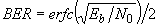#### or the Washington Post website: here.

Simulation Source Code Examples

The simulation source code comprises a test driver routine and several functions, which will be described below. This code simulates a link through an AWGN channel from data source to Viterbi decoder output.

The test driver first dynamically allocates several arrays to store the source data, the convolutionally encoded source data, the output of the AWGN channel, and the data output by the Viterbi decoder. Next. it calls the data generator, convolutional encoder, channel simulation, and Viterbi decoder functions in turn. It then compares the source data output by the data generator to the data output by the Viterbi decoder and counts the number of errors. Once 100 errors (sufficient for +/- 20% measurement error with 95% confidence) are accumulated, the test driver displays the BER for the given Es/No. The test parameters are controlled by definitions in vdsim.h .

The test driver includes a compile-time option to also measure the BER for an uncoded channel, i.e. a channel without forward error correction. I used this option to validate my Gaussian noise generator, by comparing the simulated uncoded BER to the theoretical uncoded BER given by, where Eb/N0 is expressed as a ratio, not in dB. I am happy to say that the results agree quite closely.

When running the simulations, it is important to remember the relationship between Es/N0 and Eb/N0.  As stated earlier, for the uncoded channel, Es/N0 = Eb/N0, since there is one channel symbol per bit.  However, for the coded channel, Es/N0 = Eb /N0 + 10log10(k/n).  For example, for rate 1/2 coding, Es/N0 = Eb/N0 + 10log 10(1/2) = Eb/N0 - 3.01 dB.  For rate 1/8 coding, Es/N0 = Eb/N0 + 10log 10(1/8) = Eb/N0 - 9.03 dB.

The data generator function simulates the data source. It accepts as arguments a pointer to an input array and the number of bits to generate, and fills the array with randomly-chosen zeroes and ones.

The convolutional encoder function accepts as arguments the pointers to the input and output arrays and the number of bits in the input array. It then performs the specified convolutional encoding and fills the output array with one/zero channel symbols. The convolutional code parameters are in the header file vdsim.h .

The channel simulation function accepts as arguments the desired Es/N0, the number of channel symbols in the input array, and pointers to the input and output arrays. It performs the binary (one and zero) to baseband signal level (+/- 1) mapping on the convolutional encoder channel symbol outputs. It then adds Gaussian random variables to the mapped symbols, and fills the output array. The output data are floating point numbers.

The arguments to the Viterbi decoder function are the expected Es/N0, the number of channel symbols in the input array, and pointers to its input and output arrays. First, the decoder function sets up its data structures, the arrays described in the algorithm description section. Then, it performs three-bit soft quantization on the floating point received channel symbols, using the expected E s/N0, producing integers. (Optionally, a fixed quantizer designed for a 4 dB Es/N0 can be chosen.) This completes the preliminary processing.

The next step is to start decoding the soft-decision channel symbols. The decoder builds up a trellis of depth K x 5, and then traces back to the beginning of the trellis and outputs one bit. The decoder then shifts the trellis left one time instant, discarding the oldest data, following which it computes the accumulated error metrics for the next time instant, traces back, and outputs a bit. The decoder continues in this way until it reaches the flushing bits. The flushing bits cause the encoder to converge back to state 0, and the decoder exploits this fact. Once the decoder builds the trellis for the last bit, it flushes the trellis, decoding and outputting all the bits in the trellis up to but not including the first flushing bit.

I have compiled and tested the simulation source code described above under Borland C++ Builder Version 3--please do not request help in modifying the code to compile under a different environment.  Simulation results are presented here.

Click on one of the links below to go to the beginning of that section: